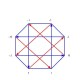# Cosmology Math Symbol “Cheat Sheet”

Cosmology Math Symbol Cheat Sheet by David Dilworth

Cosmology has quite a few math symbols. Some are familiar using standard physics notation, others are easy to confuse or “mis-remember” such as how “T” can mean either Temperature or Frequency.  Adding to that confusion, cosmology math symbols often have different meanings in other closely related fields.

So here’s a cheat sheet of common symbols used in Cosmological math. It could be helpful to Cosmology students and for those of us who have a lot of other things to remember and would like to keep our brain free for more important details. (At least that’s the excuse I’m going to use.)

If I’ve missed any vital ones, or Hubble Forbid . . . made any errors, send me a quick note and I’ll fix it as soon as I can.

Cosmology Math Symbol Cheat Sheet by David Dilworth
PS As I leave my more energetic youth behind and it gets tougher to keep 7 ideas in mind at once, I recall George Burns’ marvelous observation —

“When your memory goes . . .  forget it.”

For further reading and reference –  Here’s a list of common physics notations.

### 6 Responses to Cosmology Math Symbol “Cheat Sheet”

1.Ed Anderson says:

Thanks for the cheat-sheet. I was wondering if there’s a cosmological, or mathematical symbol for the concept of “origin”. Obviously, most search engines just start spitting out results about the “origins of mathematical symbols” which isn’t much help. What I’m looking for is something that describes the origin/perspective of an observer or assigns a context from which to execute a function or operation. Not just ‘0’ (Zero) or something similar, but a way to define the conceptual “beginning” of a given (virtual or physical) domain.

•David says:

Thank you for your question Ed.

I only grasp the outline of your idea so far.
It might help me if perhaps you could give an example?
(And I’m hoping one of our most astute readers could help.)

2.M.A. Norton says:

Orbital eccentricity? Field equations? Masses?

•David says:

However, incomplete, context free single-word sentences don’t always convey meaning.

Perhaps you could help by providing a complete question and some context so readers (including me) won’t have to guess at your meaning.

If you mean add terms for those concepts:

1. The term for Orbital eccentricity is “e” but not (yet) included as it is seldom used in cosmology.

2. The 10 “Field equations” of General Relativity are not terms, and are extensive so they do not lend themselves to this “one page cheat sheet of terms.” However, there is a link to other “Cosmology Equations” under “References, then click on “Tools.”

3. Is it really necessary to include the term for Mass as “m” ? If you think so, let me know why and you might convince me.

3.wardell lindsay says:

David,

The Math for Cosmology is Quaternions. [scalar,Vector], a 4D space, not Space Time [x+y+z,1ct] complex space.

Gravity Energy W =[c,V][,P] = [-vp.cP] =[-mGM/r.cP]

Force F= XW=[d/dr,Del][-vp,cP]

F= [vpr +cDel.P, cdP/dr -Del vp + cDelxP]eho
cdP/dr Tangent vector
-Del vp Gradient vector gravity centripetal vector
cDelxP curl/rotation vector. Gravity orbits

These terms describe the motion of Gravity motions, from Solar cystems, to Galaxies to Clusters ! The law of Gravity covers all three structures, the forces are the same.

GRT does not cover the forces and structures, Guv=8piG(Tuv + rho /\ guv)

•wardell lindsay says:

David,
corrected typos.

The Math for Cosmology is Quaternions. [scalar,Vector], a 4D space, not Space Time [x+y+z,1ct] complex space.

Gravity Energy W =[c,V][,P] = [-vp,cP] =[-mGM/r,cP]

Force F= XW=[d/dr,Del][-vp,cP]

F= [vpr + cDel.P, cdP/dr -Del vp + cDelxP]

cdP/dr Tangent vector
-Del vp Gradient vector gravity centripetal vector
cDelxP curl/rotation vector. Gravity orbits

These terms describe the motion of Gravity motions,
to Solar systems,
to Galaxies
to Clusters !

The law of Gravity covers all three structures, the forces are the same.

GRT does not cover the forces and structures, Guv=8piG(Tuv + rho /\ guv)